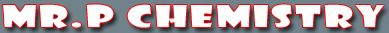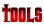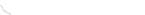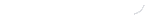activity seriesbond energybond lengthscalculatorcommon namesconversion factors: energyconversion factors: lengthconversion factors: massconversion factors: pressureconversion factors: temp.conversion factors: volumecovalent prefixesdensity solverdiatomic elementse-config. chartelement listgas law formulasKa's of polyprotic acidsKa's of weak acidsKb's of weak basesmetric conversion chartmole conversion chartmolecular geometriesorganic prefixesperiodic tableperiodic table (flash)pH/pOH converterpolyatomic ionspressure converterSI unitssolubility chartsolubility of salts rulessolubility product constantsstoichiometry charttemp. conversiontemp. formulasthermodynamic datavapor pressure of water
Elements in Crust of Earth

 The 10 Most Abundant Elements in the Earth's Crust Element Symbol Abundance by weight Oxygen O 46.10% Silicon Si 28.20% Aluminum Al 8.23% Iron Fe 5.63% Calcium Ca 4.15% Sodium Na 2.36% Magnesium Mg 2.33% Potassium K 2.09% Titanium Ti 0.565% Hydrogen H 0.14% Total 99.89%

 You may also find these related tutorials helpfulCompounds in Crust of EarthDensityElements in Crust of EarthHow To Round NumbersMetric ConversionScientific NotationSI UnitsSignificant Figure CalculationsSignificant Figures